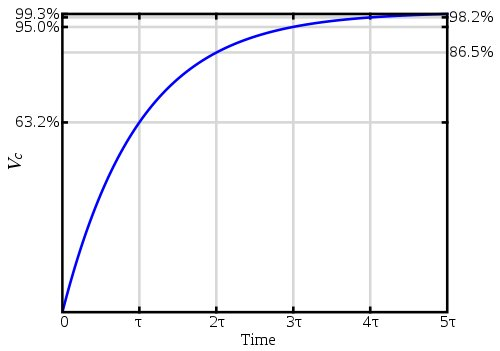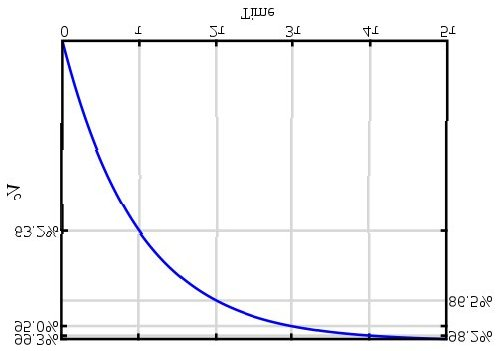Education – the RC circuit

Today we continue down the path of analog electronics theory by stopping by for an introductory look at the RC circuit. That’s R for resistor, and C for capacitor. As we know from previous articles, resistors can resist or limit the flow of current in a circuit, and a capacitor stores electric current for use in the future. And – when used together – these two simple components can be used for many interesting applications such as timing and creating oscillators of various frequencies.

How is this so? Please consider the following simple circuit:When the switch is in position A, current flows through R1 and into the capacitor C1 until it is fully charged. During this charging process, the voltage across the capacitor will change, starting from zero until fully charged, at which point the voltage will be the same as if the capacitor had been replaced by a break in the circuit – in this case 6V. Fair enough. But how long will the capacitor take to reach this state? Well the time taken is a function of several things – including the value of the resistor (R1) as it limits the flow of current; and the size of the capacitor – which determines how much charge can be stored.

If we know these two values, we can calculate the time constant of the circuit. The time constant is denoted by the character zeta (lower-case Greek Z).The time constant is the time taken (in seconds) by the capacitor C that is fed from a resistor R to charge to a certain level. The capacitor will charge to 63% of the final voltage in one time constant, 85% in two time constants, and 100% in five time constants. If you graphed the % charge against time constant, the result is exponential. That is:Now enough theory – let’s put this RC circuit to practice to see the voltage change across the capacitor as it charges. The resistor R1 will be 20k ohm, the capacitor 1000 uF.

Our time constant will be R x C which will be 20000 ohms x 0.001 farads, which equals 20 (seconds).  Notice the unit conversion – you need to go back to ohms and farads not micro-, pico- or nanofarads. So our example will take 20 seconds to reach 63% of final voltage, and 100 seconds to reach almost full voltage. This is assuming the values of the resistor and capacitor are accurate. The capacitor will have to be taken on face value as I can’t measure it with my equipment, and don’t have the data sheet to know the tolerance. The resistor measured at 19.84 k ohms, and the battery measured 6.27 volts. Therefore our real time constant should be around 19.84 seconds, give or take.

First of all, here is a shot of the little oscilloscope measuring the change in voltage over the capacitor with respect to time. The vertical scale is 1v/division:

And here is the multimeter measuring the voltage next to a stopwatch. (crude yet effective, no?)

The two videos were not the most accurate, as it was difficult to synchronise the stopwatch and start the circuit, but I hope you could see the exponential relationship between time and voltage.

What about discharging? Using the circuit above, if we moved the switch to B after charging the capacitor –  and R2 was also 20k ohm – how long would it take to discharge the capacitor? Exactly the same as charging it! So one time constant to discharge 63% and so on. So you can take the graph from above and invert it as such:How can we make use of an RC circuit?When power is applied, the capacitor starts to charge, and in doing so allows current to flow to the emitter of the transistor, which turns on the LED. However as the capacitor charges, less current passes to the base of the transistor, eventually turning it off. Therefore you can calculate time constants and experiment to create an off timer. However, a preferable way would be to make use of a 555 timer. For example, an RC combination is used to set the pulse length used in astable timing applications, for example using R1, R2 and C1:Another use of the RC circuit is oscillating. Due to varying capacitor values due to tolerance, you most likely cannot make precision frequency generators, but you can still have some fun and make useful things. Here is a classic oscillator example – an astable multivibrator:What is going on here? Here it is in action:

and here is one side being measured on the little scope:

We have two RC circuits, each controlling a transistor. When power is applied, there is no way to determine which side will start first, as this depends on the latent charge in the capacitors and the exact values of the resistors and capacitors. So to start let’s assume the left transistor (Q1) and LED are on; and the right transistor (Q2) and LED are off. The voltage at collector of Q1 will be close to zero as it is on. And the voltage at the base of Q2 will also be close to zero as C2 will initially be discharged. But C2 will now start charging via R4 and base of Q1 to around 5.4V (remember the 0.6v loss over the base-emitter junction of a transistor). While this is happening, C1 starts charging through R2. Once the voltage difference reaches 0.6V over the capacitor, Q2 is turned on.

But when Q2 is on, the voltage at the collector drops to zero, and C2 is charged, so it pulls the voltage at the base of Q1 to -5.4v, turning it off and the left LED. C1 starts charging via R1, and C2 starts charging via R3 until it reaches 0.6v. Then Q1 turns on, bringing the base of Q2 down to -5.4V – switching it off. And the whole process repeats itself. Argh. Now you can see why Arduino is so popular.

Time for a laugh – here is the result of too much current through a trimpot:

So there you have it – the RC circuit. Part of the magic of analogue electronics! And if you made it this far – check out my new book “Arduino Workshop” from No Starch Press.

In the meanwhile have fun and keep checking into tronixstuff.com. Why not follow things on twitterGoogle+, subscribe  for email updates or RSS using the links on the right-hand column? And join our friendly Google Group – dedicated to the projects and related items on this website. Sign up – it’s free, helpful to each other –  and we can all learn something.

1.Dustin J. Mitchell says:

I’m a bit confused about the multivibrator circuit. From what I can tell, the capacitors are back-biased when their corresponding transistor is on (R2 -> C1 -> Q1, for example). I implemented the circuit and checked it with a voltmeter and sure enough, it’s at around -0.5V. Isn’t this a bad idea for an electrolytic cap?

•John Boxall says:

Hello Dustin
My apologies for the delay in this reply. After speaking with a few oldies in theory, yes it is not such a good idea. However in practice we’ve been doing this for about thirty years and it’s ok due to the low power and voltage in this type of circuit – our version will run happily like this in safety. However one could always increase the resistor values and use smaller, non-polarised capacitors as well if in doubt.
Cheers, John

•Dustin J. Mitchell says:

Gotcha – thanks! I did hook this up to a ‘scope today to verify my hunch. As circuits go, it’s actually pretty complex, since the capacitors float, rather than keeping one terminal at a ground or +6V.

•John Boxall says:

Excellent! Yes, it is somewhat complex. Funnily enough, it is the standard circuit in most electronics texts here in Australia. I think there must be a lot of kids (and adults) put off learning electronics when they got to the stage of trying to figure out a multivibrator circuit. Anyhow, have fun and thanks for your input.
Cheers
John

2.John Boxall says:

Hello Mike
Very interesting, thank you for that introduction. When I update the post will look into them further.
Cheers,
John

3.Dr. Chris Shaw says:

Hi John

have tried to set up the simple off timer circuit above with C1 4.7micF, R1 100Kohm, am using a 1.5 V (or 9V) source, and a BC182L transistor. What I seem to observe is that the LEd comes on but never switches off? Would the choice of BC548 be critical in this circuit?

4.Dr. Chris Shaw says:

Hi John,

It’s ok sorted problem. Dodgy components. Circuit worked ok with 9 Volt supply so can play round with it now.

•John Boxall says:

Ah good news.

5.Yochay says:

Hi,
Very interesting article.
Is there a way to modify the oscilator, such that a single click on a switch causes only 2 or 3 blinks of the led?

6.John Boxall says:

look up high- and low-pass filters.

7.John Boxall says:

Well done, thank you for sharing this with us.

8.John Boxall says: